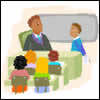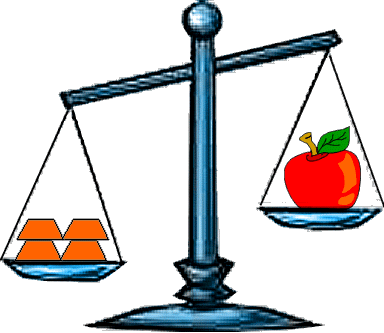#### You may also like### Back to School

Mr. Sunshine tells the children they will have 2 hours of homework. After several calculations, Harry says he hasn't got time to do this homework. Can you see where his reasoning is wrong?### The Magic Number and the Hepta-tree

Find the exact difference between the largest ball and the smallest ball on the Hepta Tree and then use this to work out the MAGIC NUMBER!### Shedding Some Light

Make an estimate of how many light fittings you can see. Was your estimate a good one? How can you decide?

# What's My Weight?

## What's My Weight?The picture above shows four equal weights on one side of the scale and an apple on the other side.

What can you say that is true about the apple and the weights?

If the apple weighs $180g$, how heavy must one weight be?

If the apple weighed $375g$, how heavy would one weight be?

If the apple was a giant one and weighed a full kilo and the weights were each $250g$, what would the scale look like? How do you know? Can you prove it?

### Why do this problem?

This problem would be useful when the group are looking at balancing weights and using grams and kilos. It requires some clear thinking and some calculation but should not take long to do.

### Key questions

Which weighs more - the apple or the weights?
How many weights are there? So how will you find out how heavy one weight is?

### Possible extension

Learners could work out similar problems when there are five weights rather than four.

### Possible support

All learners will benefit from using real scales to weight items before tackling this problem, so what is involved becomes clearer.